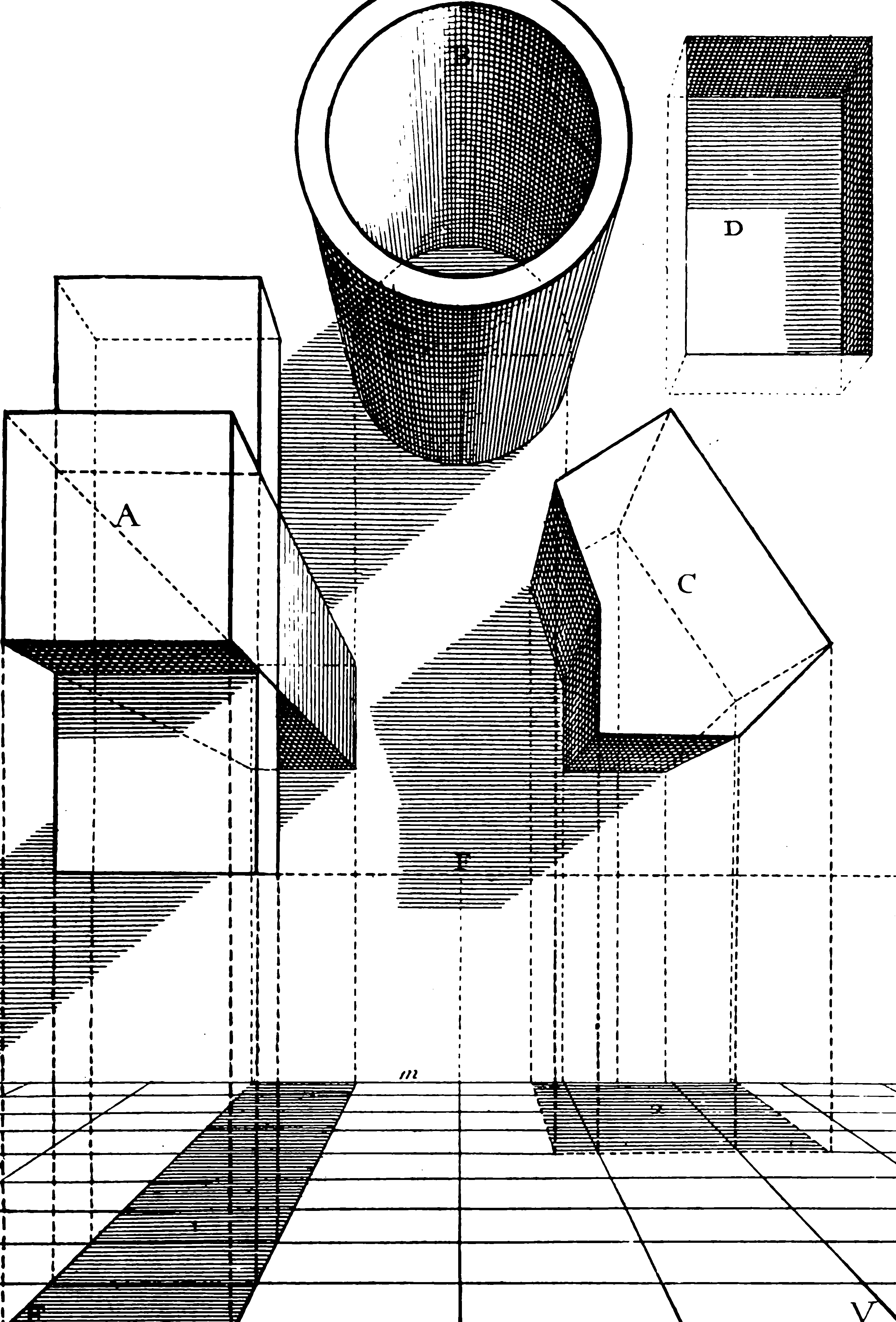# Randomized low dimensional projectionsOne way I can get at the confusing behaviours of high dimensional distributions is to instead look at low dimensional projections of them. If I have a (possibly fixed) data matrix and a random dimensional projection, what distribution does the projection have?

This idea pertains to many others: matrix factorisations, restricted isometry properties, Riesz bases, randomised regression, compressed sensing. You could also consider these results as arising from/resulting in certain structured random matrices.

## Tutorials

There is a confusing note soup, sorry. You might find it better to read a coherent overview such as Meckes’ lecture slides which include a lot of important recent developments, many of which she invented:

Related: Weird slicing problems in convex geometry. For a theoretical background as to how that relates, see Guédon (2014).

## Inner products

Djalil Chafaï introduces the The Funk-Hecke formula, also mentioned under isotropic RVs, which gives us a formula for a particularly simple case, of unit-norm RVs:

… if $$X$$ is a random vector of $$\mathbb{R}^{n}$$ uniformly distributed on $$\mathbb{S}^{n-1}$$ then for all $$y \in \mathbb{S}^{n-1}$$, the law of $$X \cdot y$$ has density $t \in[-1,1] \mapsto \frac{\Gamma\left(\frac{n}{2}\right)}{\sqrt{\pi} \Gamma\left(\frac{n-1}{2}\right)}\left(1-t^{2}\right)^{\frac{n-3}{2}}$ This law does not depend on the choice of $$y \in \mathbb{S}^{n-1}$$. It is symmetric in the sense that $$X \cdot y$$ and $$-(X \cdot y)$$ have same law. The law of $$|X \cdot y|$$ is the image of the law $$\operatorname{Beta}\left(\frac{3}{2}, \frac{n-1}{2}\right)$$ by the map $$u \mapsto \sqrt{u}$$. The law of $$X \cdot y$$ is

• if $$n=2$$ : an arcsine law,
• if $$n=3$$ : a uniform law (Archimedes principle),
• if $$n=4$$ : a semicircle law.

whuber asserts that $$(X \cdot y+1)\sim\operatorname{Beta}((d-1)/2,(d-1)/2),$$ and $$\operatorname{Var}(X \cdot y)=1/d.$$

## Random projections are kinda Gaussian

More generally things are not so exact. But they are still reasonably nice, in that there are lots of tasty limit theorems with nice regular behaviour.

A classic introductory concept: Diaconis-Freedman effect. Diaconis and Freedman (1984) show that (under some mild omitted conditions), $\left\{x_{1}, \ldots, x_{n}\right\} \subseteq \mathbb{R}^{d}$ is a data set (possibly deterministic with no assumption on generating process), $$\theta$$ is a uniform random point in the sphere $$\mathbb{S}^{d-1},$$ and $\mu_{x}^{\theta}:=\frac{1}{n} \sum_{i=1}^{n} \delta_{\left\langle x_{i}, \theta\right\rangle}$ is the empirical measure of the projection of the $$x_{i}$$ onto $$\theta$$, then as $$n, d \rightarrow \infty,$$ the measures $$\mu_{x}^{\theta}$$ tend to $$\mathcal{N}\left(0, \sigma^{2}\right)$$ weakly in probability. This succinct statement is modeled on Elizabeth Meckes'.

A lesson is that even non-Gaussian, non-independent data can become nearly i.i.d. Gaussian in low dimensional projection, as Dasgupta, Hsu, and Verma (2006) argue in their introduction.

This has been taken to incredible depth in the work of Elizabeth Meckes 1980—2020 whose papers serve as the canonical textbook in the area for now. Two foundational ones are Chatterjee and Meckes (2008) and E. Meckes (2009) and there is a kind of user guide in E. Meckes (2012b) which leverages Stein’s method a whole bunch.

## Random projections are distance preservingWhat makes random embeddings go. The most famous result is the Johnson-Lindenstrauss lemma.

A simple proof of that is given by Dasgupta and Gupta (2003)

• “Locality-Sensitive Hashing (LSH) is an algorithm for solving the approximate or exact Near Neighbor Search in high dimensional spaces.” (Is this random even?)

• John Myles White explains why the Johnson-Lindenstrauss lemma is worth knowing

## Projection statistics

Another key phrase we can look for is probability on the Stiefel manifold, which is a generalization of a familiar concept from random orthonormal matrices. Stiefel manifolds generalise an orthonormal matrix because they can map between spaces of different dimension. Formally, the Stiefel manifold $$V_{k, m}$$ is the space of $$k$$ frames in the $$m$$ -dimensional real Euclidean space $$R^{m},$$ represented by the set of $$m \times k$$ matrices $$X$$ such that $$X^{\prime} X=I_{k},$$ where $$I_{k}$$ is the $$k \times k$$ identity matrix. There are some interesting cases in low dimensional projections served by $$k\ll m$$ especially $$k=1.$$

Cool results in this domain are, e.g. Chikuse and 筑瀬 (2003);E. S. Meckes and Meckes (2013);E. Meckes (2012a);Stam (1982).

General projections results are in Dümbgen and Del Conte-Zerial (2013).

An important trick is the distribution of isotropic unit vectors.

Let $$Z=Z^{(q)}:=\left(Z_{1}, Z_{2}, \ldots, Z_{d}\right)$$ be a random matrix in $$\mathbb{R}^{m \times k}$$ with independent, standard Gaussian column vectors $$Z_{j} \in \mathbb{R}^{m} .$$ Then $\Theta:=Z\left(Z^{\top} Z\right)^{-1 / 2}=Z/\|Z\|_2^2$ has the desired distribution, and $\Theta=m^{-1 / 2} Z\left(I+O_{p}\left(m^{-1 / 2}\right)\right) \quad \text { as } m \rightarrow \infty.$

Vershynin’s writing on a variety of hard high-dimensional probability results is pretty accessible: Vershynin (2015);Vershynin (2018). These bleed over into concentration results.

I wrote one of my own… TBD.

## Concentration theorems for projections

Many, e.g. Dasgupta, Hsu, and Verma (2006);Dümbgen and Del Conte-Zerial (2013);Gantert, Kim, and Ramanan (2017);Kim, Liao, and Ramanan (2020).

### No comments yet. Why not leave one?

GitHub-flavored Markdown & a sane subset of HTML is supported.